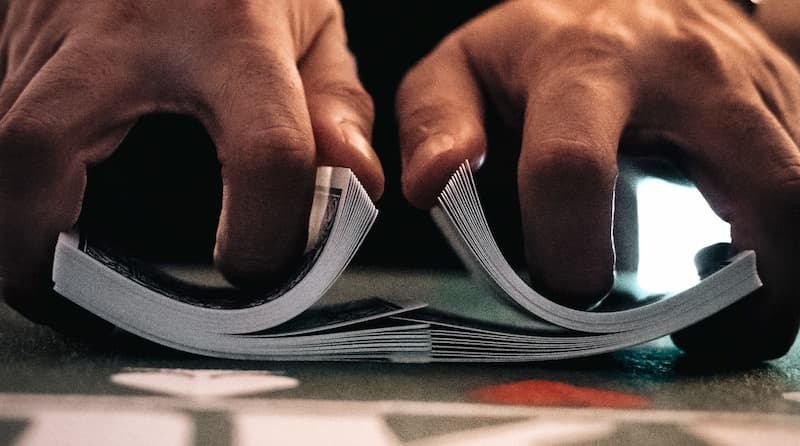# Shuffle productThe shuffle product of two words, w1 and w2, written

w1 Ш w2,

is the set of all words formed by the letters in w1 and w2, preserving the order of each word’s letters. The name comes from the analogy with doing a riffle shuffle of two decks of cards.

For example, bcd Ш ae, the shuffle product of bcd and ae, would be all permutations of abcde in which the consonants appear in alphabetical order and the vowels are also in alphabetical order. So abecd and baecd would be included, but badec would not be because the d and c are in the wrong order.

## Side note on Ш

Incidentally, the symbol for shuffle product is the Cyrillic letter sha (Ш, U+0428), the only Cyrillic letter commonly used in mathematics, at least internationally. Presumably Russian mathematicians use other Cyrillic letters, but the only Cyrillic letter an American mathematician, for example, is likely to use is Ш.

The uses of Ш that I’m aware of are the Dirac comb distribution, the Tate–Shafarevich group, and the shuffle product.

## Duplicate letters

What is the shuffle product of words containing duplicate letters? For example, what about the shuffle product of bread and crumb? Each word contains an r. The shuffle product, defined above as a set, doesn’t distinguish between the two rs. But another way to define the shuffle product is as a formal sum, with coefficients that count duplicates.

Imagine coloring the letters in abc blue and the letters in cde red. Then abccde and abccde would count as two different possibilities, one with blue c followed by red c, and one the other way around. This term in the formal sum would be 2abccde, the two capturing that there are two ways to arrive at this word.

You could also have duplicate letters within a single word. So in banana, for example, you could imagine coloring each a a different color and coloring the two ns different colors.

## Mathematica code

```shuffleW[s1_, s2_] :=
Module[{p, tp, ord},
p = Permutations@Join[1 & /@ s1, 0 & /@ s2]\[Transpose];
tp = BitXor[p, 1];
ord = Accumulate[p] p + (Accumulate[tp] + Length[s1]) tp;
Outer[Part, {Join[s1, s2]}, ord, 1][]\[Transpose]]
```

This code takes two lists of characters and returns a list of lists of characters. You can use this to compute both senses of the shuffle product. For example, let’s compute abc Ш ac.

The Mathematica command

`    shuffleW[{a, b, c}, {a, c}]`

returns a list of 10 lists:

```
{{a, b, c, a, c}, {a, b, a, c, c}, {a, b, a, c, c},
{a, a, b, c, c}, {a, a, b, c, c}, {a, a, c, b, c},
{a, a, b, c, c}, {a, a, b, c, c}, {a, a, c, b, c},
{a, c, a, b, c}}
```

If we ask for the union of the set above with `Union[%]` we get

```    {{a, a, b, c, c}, {a, a, c, b, c}, {a, b, a, c, c},
{a, b, c, a, c}, {a, c, a, b, c}}
```

So using the set definition, we could say

abc Ш ac = {aabcc, aacbc, abacc, abcac, acabc}.

Using the formal sum definition we could say

abc Ш ac = 4aabcc + 2aacbc + 2abacc + abcacacabc.

## Related posts

Photo by Amol Tyagi on Unsplash

## 5 thoughts on “Shuffle product”

1. Also, Jeffrey Shallit uses Ш for the perfect shuffle of two words of equal length: clipШaloe = calliope.

2. Dave Pawson

Of course you would use Mathematica!
Amusing, of use? I’m sat here wondering why (and can visualise 6 answers!).
Thank you John

3. Yes, the shuffle product comes up in applications. I’ve seen it used in combinatorial problems.

4. The letter Sha is, actually, the Hebrew Shin (ש).
Add this to the Hebrew Aleph (א), also in regular use…

5. The consensus is that Sha probably derived from Shin, but some think it may have derived from Coptic Shai. Then you can ask where Coptic got its letter Shai from. Maybe from Hebrew Shin?